GATE  >  GATE Numerical Ability Questions (Quant & Analytical) -1

# GATE Numerical Ability Questions (Quant & Analytical) -1

Test Description

## 10 Questions MCQ Test | GATE Numerical Ability Questions (Quant & Analytical) -1

GATE Numerical Ability Questions (Quant & Analytical) -1 for GATE 2023 is part of GATE preparation. The GATE Numerical Ability Questions (Quant & Analytical) -1 questions and answers have been prepared according to the GATE exam syllabus.The GATE Numerical Ability Questions (Quant & Analytical) -1 MCQs are made for GATE 2023 Exam. Find important definitions, questions, notes, meanings, examples, exercises, MCQs and online tests for GATE Numerical Ability Questions (Quant & Analytical) -1 below.
Solutions of GATE Numerical Ability Questions (Quant & Analytical) -1 questions in English are available as part of our course for GATE & GATE Numerical Ability Questions (Quant & Analytical) -1 solutions in Hindi for GATE course. Download more important topics, notes, lectures and mock test series for GATE Exam by signing up for free. Attempt GATE Numerical Ability Questions (Quant & Analytical) -1 | 10 questions in 15 minutes | Mock test for GATE preparation | Free important questions MCQ to study for GATE Exam | Download free PDF with solutions
 1 Crore+ students have signed up on EduRev. Have you?
GATE Numerical Ability Questions (Quant & Analytical) -1 - Question 1

### The number of Hens, Ducks, and Goats in farm P are 65, 91 and 169, respectively. The total number of Hens, Ducks and Goats in a nearby farm Q is 416. The ratio of hens : ducks : goats in farm Q is 5 : 14 : 13. All the hens, ducks and goats are sent from farm Q to farm P. The new ratio of hens : ducks : goats in farm P is ____________.

Detailed Solution for GATE Numerical Ability Questions (Quant & Analytical) -1 - Question 1

In Farm P:

Hens = 65, Ducks = 91 and Goats = 169

In Farm Q:

Total number of Hens, Ducks, and Goats = 416

The ratio of Hens : Ducks : Goats = 5 : 14 : 13

Total number of Hens = 5/32 × 416 = 65
Total number of Ducks = 14/32 × 416 = 182
Total numbers of Goats = 13/32 × 416=169

From Farm P, all the Hens, Ducks and Goats are sent to Farm Q.

Total Hens in farm Q = 65 + 65 = 130

Total Ducks in farm Q = 91 + 182 = 273

Total Goats in farm Q = 169 + 169 = 338

New Ratio = 130 : 273 : 338 = 10 : 21 : 26

GATE Numerical Ability Questions (Quant & Analytical) -1 - Question 2

### Find the missing sequence in the letter series. B, FH, LNP, __________.

Detailed Solution for GATE Numerical Ability Questions (Quant & Analytical) -1 - Question 2

The series formed is as follows,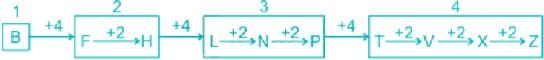GATE Numerical Ability Questions (Quant & Analytical) -1 - Question 3

### A jigsaw puzzle has 2 pieces. One of the pieces is shown above. Which one of the given options for the missing piece when assembled will form a rectangle? The piece can be moved, rotated or flipped to assemble with the below piece.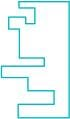Detailed Solution for GATE Numerical Ability Questions (Quant & Analytical) -1 - Question 3

Draw a rectangle and carved out the remaining position.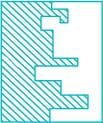After the carved-out portion is out, make a mirror image of it.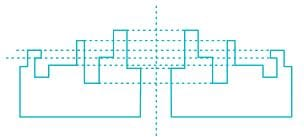GATE Numerical Ability Questions (Quant & Analytical) -1 - Question 4

If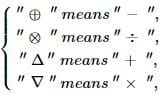then, the value of the expression Δ 2 ⊕ 3 Δ ((4 ⊗ 2) ∇ 4) =

Detailed Solution for GATE Numerical Ability Questions (Quant & Analytical) -1 - Question 4

Concept:

Replace the symbol as per their standard sign and solve using BODMAS.

Calculation:

Given:

Δ 2 ⊕ 3 Δ ((4 ⊗ 2) ∇ 4) = + 2 - 3 + ((4 ÷ 2) × 4)

∴ + 2 - 3 + (2 × 4)

∴ + 2 - 3 + 8

∴ 10 - 3 = 7

GATE Numerical Ability Questions (Quant & Analytical) -1 - Question 5

The distribution of employees at the rank of executives, across different companies C1, C2, ..., C6 is presented in the chart given above. The ratio of executives with a management degree to those without a management degree in each of these companies is provided in the table above. The total number of executives across all companies is 10,000.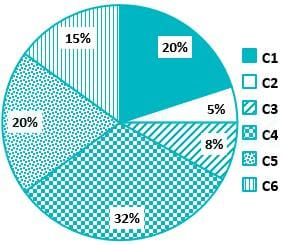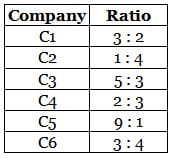Q. The total number of management degree holders among the executives in companies C2 and C5 together is _____.

Detailed Solution for GATE Numerical Ability Questions (Quant & Analytical) -1 - Question 5

Concept:

From pie-chart, we can get the total number of executives in each company.

From the ratio table, we can get the numbers of executives with and without a management degree.

Calculation:

Given:

Total executives = 10,000

Percentage of executives in C2 = 5%, C5 = 20%

Ratio of executives with and without management degree C2 = 1 : 4, and C5 = 9 : 1.

Executives in C2 = 5/100 × 10000 = 500

Executives in C5 = 20/100 × 10000 = 2000

Executives with Management degree holders in C2 = 1/5 × 500 = 100

Executives with Management degree holders in C5 = 9/10 × 2000=1800

∴ total executives with management degree holders in C2 and C5 = 100 + 1800 = 1900.

GATE Numerical Ability Questions (Quant & Analytical) -1 - Question 6

A digital watch X beeps every 30 seconds while watch Y beeps every 32 seconds.They beeped together at 10 AM.The immediate next time that they will beep together is _____.

Detailed Solution for GATE Numerical Ability Questions (Quant & Analytical) -1 - Question 6

Concept:

The Watched will beep together at the time of their Least Common Multiple (LCM).

Calculation:

Given:

Watch X beeps at = 30 sec

Watch Y beeps at = 32 sec

They beep together at = 10.00 AM

LCM of (30 & 32) = 480 sec

And X and Y will beep together after 480 sec or 8 minutes.

So Next beep time = 10.08 AM

GATE Numerical Ability Questions (Quant & Analytical) -1 - Question 7

The front door of Mr X's house faces East. Mr X leaves the house, walking 50 m straight from the back door that is situated directly opposite to the front door. He then turns to his right, walks for another 50 m and stops. The direction of the point Mr X is now located at with respect to the starting point is ______.

Detailed Solution for GATE Numerical Ability Questions (Quant & Analytical) -1 - Question 7

Tracing path followed by Mr. X,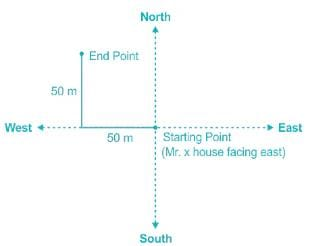Hence Mr. X will be in North-West direction from the starting point.

GATE Numerical Ability Questions (Quant & Analytical) -1 - Question 8

Given below are two statements 1 and 2, and two conclusions I and II.

Statement 1: All entrepreneurs are wealthy.

Statement 2: All wealthy are risk seekers.

Conclusion I: All risk seekers are wealthy.

Conclusion II: Only some entrepreneurs are risk seekers.

Based on the above statements and conclusions, which one of the following options is CORRECT?

Detailed Solution for GATE Numerical Ability Questions (Quant & Analytical) -1 - Question 8

The Venn diagram according to the given statements is as follows: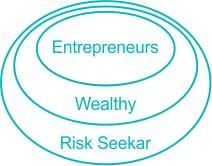Conclusions:

All risk seekers are wealthy. → False (All wealthy are risk seekers but can't say vice versa is also true)

Only some entrepreneurs are risk seekers → False (All entrepreneurs are wealthy and All wealthy are risk seekers so all entrepreneurs will be risk seekers)

Hence neither conclusion I nor II is correct.

GATE Numerical Ability Questions (Quant & Analytical) -1 - Question 9

The ratio of the area of the inscribed circle to the area of the circumscribed circle of an equilateral triangle is _____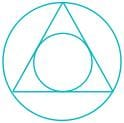Detailed Solution for GATE Numerical Ability Questions (Quant & Analytical) -1 - Question 9

Let the radius of the circumscribed circle is R, and the radius of the inscribed circle is r.

Then area of circumscribed circle = πR2

Then the area of the inscribed circle = πr2

We know the angle between two adjacent sides in an equilateral triangle is 60°.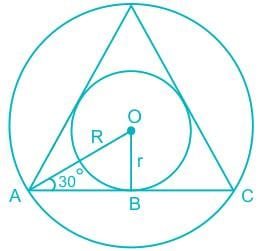ΔOAB, Sin30 = r / R

R = 2r

The ratio of the area of the inscribed circle to the area of the circumscribed circle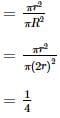GATE Numerical Ability Questions (Quant & Analytical) -1 - Question 10

A box contains 15 blue balls and 45 black balls. If 2 balls are selected randomly, without replacement, the probability of an outcome in which the first selected is a blue ball and the second selected is a black ball, is ______.

Detailed Solution for GATE Numerical Ability Questions (Quant & Analytical) -1 - Question 10

Concept:

P(E) = Number of favorable outcomes / Total number of outcomes

Calculation:

Given:

Number of blue balls = 15, Number of black balls = 45

Total number of balls = 15 + 45 = 60 balls

Balls are drawn from the box at random without replacement.

The probability of drawing first blue ball and second black ball is;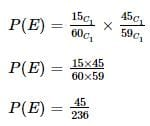Use Code STAYHOME200 and get INR 200 additional OFF Use Coupon Code
Information about GATE Numerical Ability Questions (Quant & Analytical) -1 Page
In this test you can find the Exam questions for GATE Numerical Ability Questions (Quant & Analytical) -1 solved & explained in the simplest way possible. Besides giving Questions and answers for GATE Numerical Ability Questions (Quant & Analytical) -1, EduRev gives you an ample number of Online tests for practice